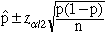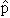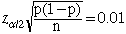HI my name is Charles and the level of the question is equivalent a college level course. I am a student and have a statistics question which has given me some difficulty. Heres the question: The U.S Transportation Dept. will randomly sample traffic reports to estimate the proportion of accidents involving people over the age of 70. The Dept. has no advance estimate of this proportion. how many reports should the dept select to be atleast 97% confident that the estimate is within .01 of the true proportion? i thought about using the interval of confidence but i dont know the mean of Xbar or the sample stand. deviation. I also have to find out the sample size but i tried this and didnt know how to find (d) in the equation. please email me back the answer and the way to do it with the different variables and equations with some explanation--thanks Hi Charles, The goal of the study is to produce a confidence interval estimate of a proportion. The expression for the endpoints of a confidence interval for a proportion is given byWhere p is the true proportion,is its estimated value, n is the sample size and zalpha/2 is the appropriate z-value. (When calculating the endpoints of the confidence interval, p is estimated by.) In the sample size problem that you have, you can find the appropriate z-value for a 97% confidence interval, and since you want the estimate to be within 0.01 of the true proportion you know thatHence if you knew p, you could solve for n. Different values for p will, of course, give different values for n. What is known is that if p = 1/2 then n attains the largest value possible. Thus if you have no previous estimate for p, let p = 1/2 and solve for n. This will give a sample size that is large enough to ensure that the estimate is within .01 of the true proportion. The sample size may, however, be much larger than you need. I hope this helps, Harley Go to Math Central